# Timeseries#

In many domains it is common to plot scalar values as a function of time (or other single dimensions). As long as the total number of datapoints is relatively low (in the tens of thousands, perhaps) and there are only a few separate curves involved, most plotting packages will do well. However, for longer or more frequent sampling, you’ll be required to subsample your data before plotting, potentially missing important peaks or troughs in the data. And even just a few timeseries visualized together quickly run into highly misleading overplotting issues, making the most recently plotted curves unduly prominent.

For applications with many datapoints or when visualizing multiple curves, datashader provides a principled way to view all of your data. In this example, we will synthesize several time-series curves so that we know their properties, and then show how datashader can reveal them.

```import datetime
import pandas as pd
import numpy as np
import xarray as xr
```

## Create some fake timeseries data#

Here we create a fake time series signal, then generate many noisy versions of it. We will also add a couple of “rogue” lines, with different statistical properties, and see how well those stand out from the rest.

```# Constants
np.random.seed(2)
n = 100000                               # Number of points
cols = list('abcdefg')                   # Column names of samples
start = datetime.datetime(2010, 10, 1, 0)   # Start time

# Generate a fake signal
signal = np.random.normal(0, 0.3, size=n).cumsum() + 50

# Generate many noisy samples from the signal
noise = lambda var, bias, n: np.random.normal(bias, var, n)
data = {c: signal + noise(1, 10*(np.random.random() - 0.5), n) for c in cols}

# Add some "rogue lines" that differ from the rest
cols += ['x'] ; data['x'] = signal + np.random.normal(0, 0.02, size=n).cumsum() # Gradually diverges
cols += ['y'] ; data['y'] = signal + noise(1, 20*(np.random.random() - 0.5), n) # Much noisier
cols += ['z'] ; data['z'] = signal # No noise at all

# Pick a few samples from the first line and really blow them out
locs = np.random.choice(n, 10)
data['a'][locs] *= 2

# Create a dataframe
data['Time'] = [start + datetime.timedelta(minutes=1)*i for i in range(n)]

df = pd.DataFrame(data)
df.tail()
```
a b c d e f g x y z Time
99995 -43.266888 -51.619471 -42.867714 -45.723507 -47.229012 -44.246641 -46.509765 -54.598808 -44.922483 -48.523868 2010-12-09 10:35:00
99996 -42.733578 -51.773237 -42.984615 -45.269990 -45.537120 -45.258385 -44.763207 -54.623914 -45.116631 -48.523325 2010-12-09 10:36:00
99997 -42.614768 -51.268256 -42.201864 -45.497689 -46.426903 -45.170198 -45.393405 -53.837847 -44.981804 -47.741589 2010-12-09 10:37:00
99998 -44.684017 -50.131985 -42.204521 -45.856321 -48.606589 -45.880804 -46.653791 -53.910759 -43.639239 -47.806325 2010-12-09 10:38:00
99999 -46.027779 -50.496361 -42.615223 -45.025591 -46.462669 -43.848973 -47.319626 -54.216269 -45.546920 -48.076312 2010-12-09 10:39:00

The native datashader API illustrated here does not support datetimes directly so we create a new column casting the datetimes to integer:

```df['ITime'] = pd.to_datetime(df['Time']).astype('int64')
```

Now we can compute the x- and y-ranges:

```# Default plot ranges:
x_range = (df.iloc.ITime, df.iloc[-1].ITime)
y_range = (1.2*signal.min(), 1.2*signal.max())

print("x_range: {0} y_range: {1}".format(x_range,y_range))
```
```x_range: (1285891200000000000, 1291891140000000000) y_range: (-104.8094774985483, 100.09816883332905)
```

## Plotting all the datapoints#

With datashader, we can plot all the datapoints for a given timeseries. Let’s select the first curve ‘a’ and draw it into an aggregate grid, connecting each datapoint in the series:

```%%time
cvs = ds.Canvas(x_range=x_range, y_range=y_range, plot_height=300, plot_width=900)
aggs= dict((c, cvs.line(df, 'ITime', c)) for c in cols)
```
```CPU times: user 13.2 s, sys: 69 ms, total: 13.3 s
Wall time: 13.3 s
```
```img
```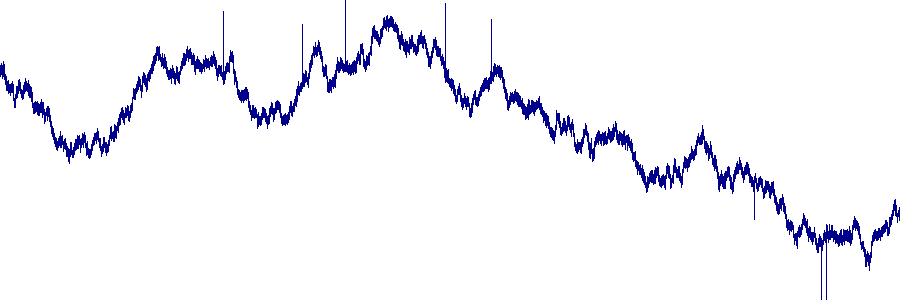The result looks similar to what you might find in any plotting program, but it uses all 100,000 datapoints, and would work similarly for 1, 10, or 100 million points (determined by the `n` attribute above).

Why is using all the datapoints important? To see, let’s downsample the data by a factor of 10, plotting 10,000 datapoints for the same curve:

```mask = (df.index % 10) == 0
```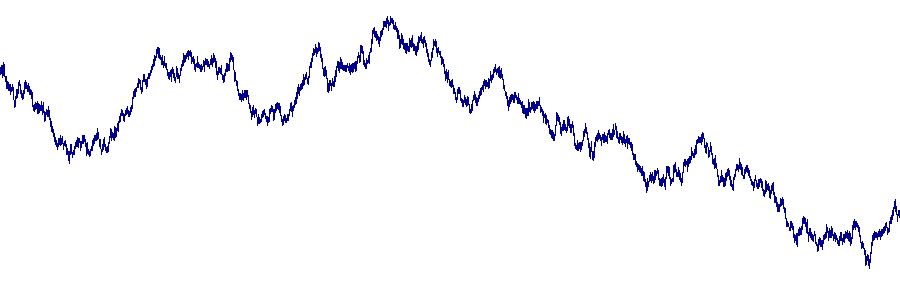The resulting plot is similar, but now none of the “blown up” datapoints (sharp spikes) that were clearly visible in the first version are visible at all! They didn’t happen to be amongst the sampled points, and thus do not show up in this plot, which should never be a problem using datashader with all the points.

## Antialiasing#

With the default `ds.count()` aggregator, `Canvas.line()` uses a very simple representation for a line (strictly, a polyline, i.e., a connected set of line segments). This representation has a clear mathematical interpretation: each pixel’s count is incremented by 1 when a line segment passes through that pixel. Having such a clear interpretation is useful when summing large numbers of curves, as illustrated in later sections below. However, the result will have sharp transitions between pixels, giving it a blocky appearance, and so if you prefer a smoother rendering where the count is spread between multiple pixels, or if you simply want to specify a thicker line for visibility, you can pass a `line_width` greater than zero and Datashader will draw a (potentially thicker) line and antialias it with intermediate values:

```cvs2  = ds.Canvas(x_range=(12879023 * 1E11, 12879070 * 1E11),
y_range=(37, 50), plot_height=200, plot_width=500)

w0   = tf.shade(cvs2.line(df, 'ITime', 'a', line_width=0), name="line_width 0")
w1   = tf.shade(cvs2.line(df, 'ITime', 'a', line_width=1), name="line_width 1")
w5   = tf.shade(cvs2.line(df, 'ITime', 'a', line_width=5), name="line_width 5")

tf.Images(w0, w1, w5).cols(1)
```
 line_width 0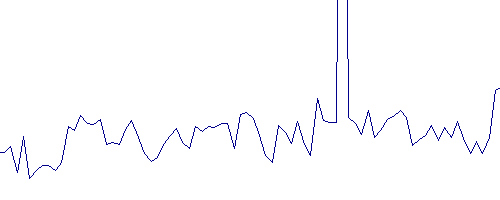line_width 1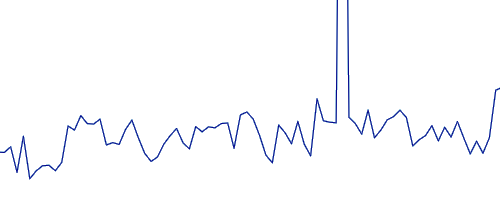line_width 5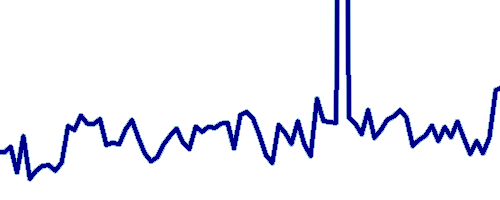Antialiasing and using large widths does slow down the rendering and may make it more difficult to interpret large collections of line segments, so it is not on by default.

## Overplotting problems#

What happens if we overlay multiple curves? In a traditional plotting program, there would be serious issues with overplotting, because these curves are highly overlapping. To show what would typically happen, let’s brute-force merge the images corresponding to each of the curves:

```renamed = [aggs[key].rename({key: 'value'}) for key in aggs]
merged = xr.concat(renamed, 'cols')
```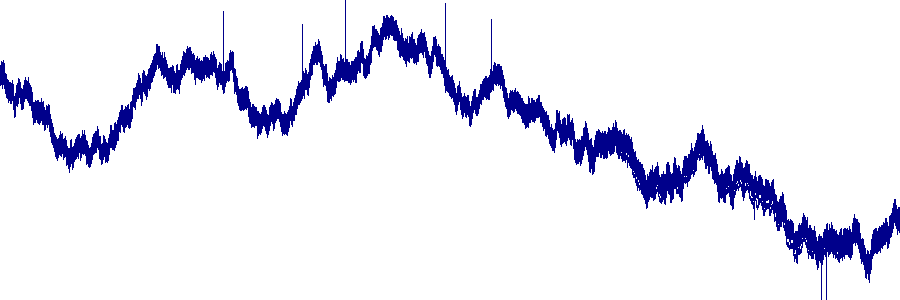The `any` operator merges all the data such that any pixel that is lit up for any curve is lit up in the final result. Clearly, it is difficult to see any structure in this fully overplotted data; all we can see is the envelope of these curves, i.e. the minimum and maximum value of any curve for any given time point. It remains completely unclear how the various curves in the set relate to each other, or even how many curves are being plotted here. We already know that we put in one particularly noisy curve, which presumably determines the envelope, but there’s no way to tell that from the plot.

We can of course try giving each curve a different color:

```colors = ["red", "grey", "black", "purple", "pink",
"yellow", "brown", "green", "orange", "blue"]
imgs = [tf.shade(aggs[i], cmap=[c]) for i, c in zip(cols, colors)]
tf.stack(*imgs)
```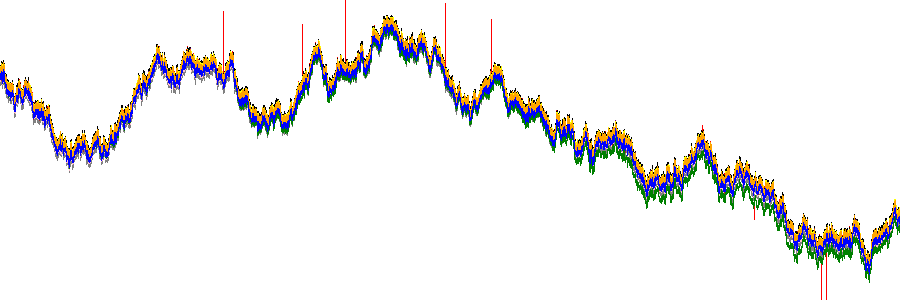But that doesn’t help much; there are 10 curves, but only three or four colors are visible, due to overplotting. Problems like that will just get much worse if there are 100, 1000, or 1 million curves. Moreover, the results will look entirely different if we plot them in the opposite order:

```tf.stack(*reversed(imgs))
```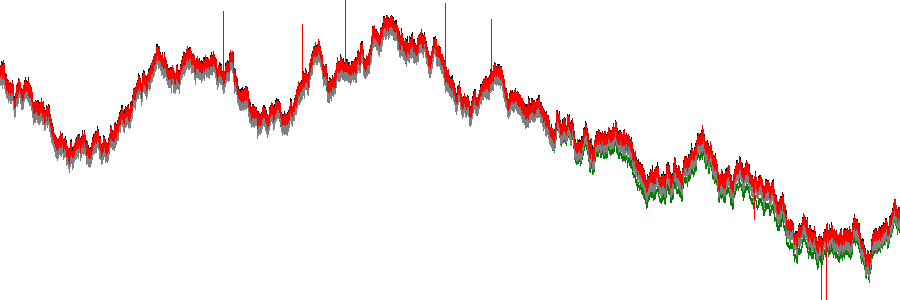Having the visualization look completely different for an arbitrary choice like the plotting order is a serious problem if we want to understand the properties of this data, from the data itself.

## Highlighting specific curves#

The data set also includes a couple of traces that are difficult to detect in the `.sum()` plot above, one with no noise and one with much higher noise. One way to detect such issues is to highlight each of the curves in turn, and display it in relation to the datashaded average values. For instance, those two curves (each highlighted in red below) stand out against the pack as having less or more noise than is typical:

```tf.stack(total, tf.shade(aggs['z'], cmap=["lightblue", "red"]))
```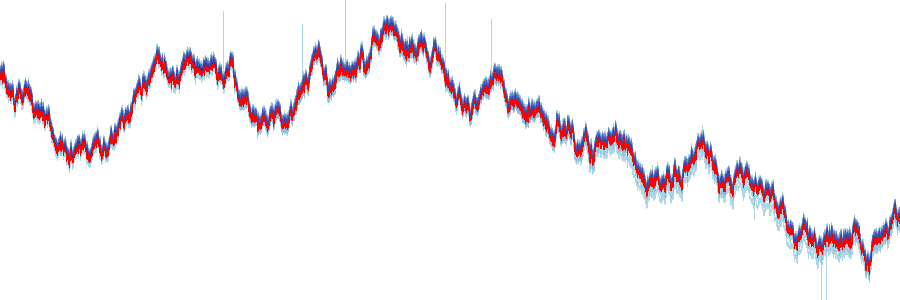```tf.stack(total, tf.shade(aggs['y'], cmap=["lightblue", "red"]))
```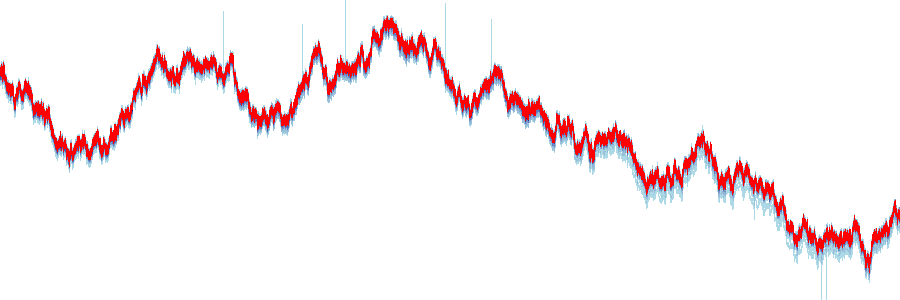## Area plots#

As an alternative to plotting time-series data as a line, the same data can be plotted as a filled area plot. This approach serves to emphasise whether the time series has a positive or negative sign. Here is an example of a filled area plot for the ‘a’ curve.

```cvs = ds.Canvas(x_range=x_range, y_range=y_range, plot_height=300, plot_width=900)
agg = cvs.area(df, x='ITime', y='a')
img
```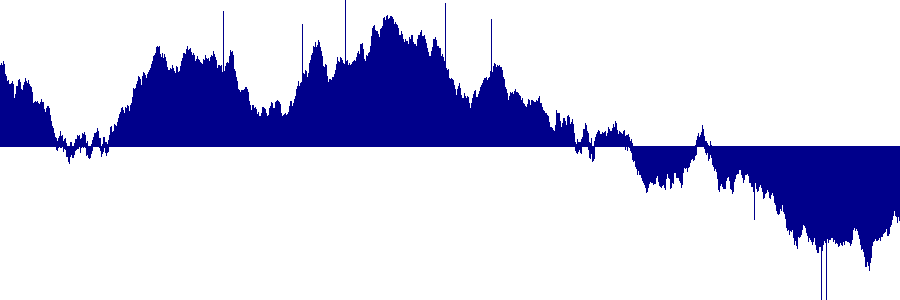By specifying the y_stack argument, an area plot can also be used to display the difference between two curves. Here is an example of an area plot of the difference between the ‘a’ and ‘b’ curves

```cvs = ds.Canvas(x_range=x_range, y_range=y_range, plot_height=300, plot_width=900)
agg = cvs.area(df, x='ITime', y='a', y_stack='b')
img
```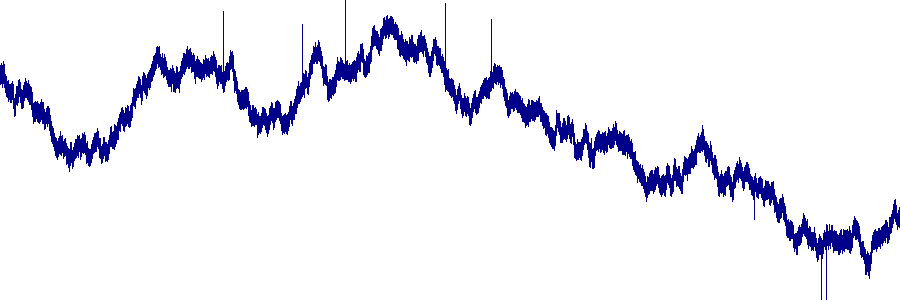## Dynamic Plots#

In practice, it might be difficult to cycle through each of the curves to find one that’s different, as done above. Perhaps a criterion based on similarity could be devised, choosing the curve most dissimilar from the rest to plot in this way, which would be an interesting topic for future research. In any case, one thing that can be achieved with HoloViews is to make the plot fully interactive, with direct support for datetimes so that the viewer can zoom in and discover such patterns dynamically with correctly formatted axes.

```import holoviews as hv```opts = hv.opts.RGB(width=600, height=300)